View Course Path

# VHDL code for all logic gates using dataflow method – full code and explanation

Logic gates are the building blocks of digital electronics. Digital electronics employ boolean logic. And logic gates are the physical circuits that allow boolean logic to manifest in the real world.

In this post, we will take a look at implementing the VHDL code for all logic gates using dataflow architecture. First, we will take a look at the logic equations of all the gates and then the syntax. We will also test our logic by writing a testbench. In addition to that, we will generate an RTL schematic to look at the circuit that our VHDL code created. Finally, we will generate a simulation waveform for final verification.

Contents

## Explanation of the VHDL code for all logic gates using dataflow method. How does the code work?

This is the first VHDL program in our VHDL course. And thus it’s an easy one. Since we are using the dataflow modeling architecture to implement all the logic gates, all we need are the logic diagrams and the logic equations of all the gates.

This program will help us understand how to declare input and output ports in a VHDL program. It will also show us the implementation of the assignment operator.

To assign a particular port to a certain value in VHDL, we use an assignment operator.

target <= source

### Logic diagram and logic equation of AND gate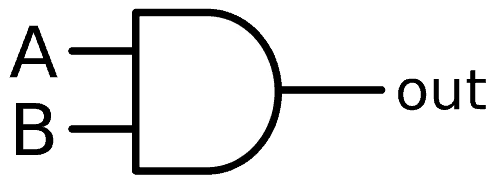AND gates have two inputs and one output, and they implement the boolean logic of multiplication. Its equation is as follows:

Y(A and B) = A.B

### Logic diagram and logic equation of NAND gate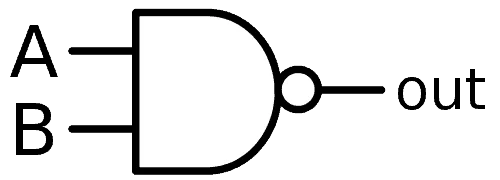NAND gates have two inputs and one output and implement the inverse boolean logic of multiplication. Its equation is as follows:

Y (A nand B) =$\overline {A.B}$ = A|B =$A\uparrow B$

### Logic diagram and logic equation of OR gate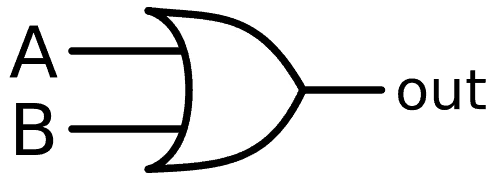OR gates have two inputs and one output, and they implement the boolean logic of addition. Its equation is as follows:

Y (A or B) = A + B

### Logic diagram and logic equation of NOR gate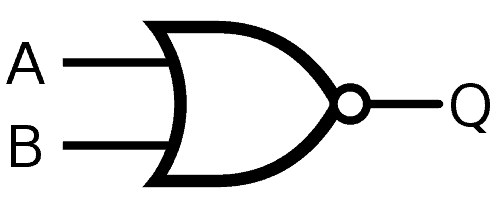NOR gates have two inputs and one output, and they implement the inverse boolean logic of addition. Its equation is as follows:

Y (A nor B) =$\overline {A+B}$ =$A\downarrow B$

### Logic diagram and logic equation of NOT gateNOT gates have one input and one output, and it implements the boolean logic of inversion. It is an inverter. Its equation is as follows:

Y (not A) = A’ =$\overline { A }$

### Logic diagram and logic equation of XOR gateXOR gates have two inputs and one output, and they implement the special boolean logic of inequality detection. The EXOR gate gives a high output every time it detects an inequality in the inputs. Its equation is as follows:

Y (A exor B) = A$\oplus$B = AB’ + A’B

### Logic diagram and logic equation of XNOR gateXNOR gates have two inputs and one output, and they implement the special boolean logic of equality detection. The EXNOR gate gives a high output every time it detects equality in the inputs. Its equation is as follows:

Y (A exnor B) =$\overline {A \oplus B}$ = A’B’ + AB

Now that we have the logic equations of all the gates, we can begin writing the code by first declaring the architecture-entity pair. Here we will show that the architecture we are following is dataflow, and we will declare our input and output ports.

We are naming our entity as ALLGATES_SOURCE.

You can name it anything and also use the underscore symbol. This is just for better file management. We will apply the same inputs to all the logic gates and take separate outputs from each of them. So the syntax will be like this:

entity ALLGATES_SOURCE is
Port ( A,B : in  STD_LOGIC;
P, Q, R, S, T, U, V : out  STD_LOGIC);
end ALLGATES_SOURCE;

architecture dataflow of ALLGATES_SOURCE is

begin

Next, all that is left to do is assign the outputs to their respective boolean expressions for each logic gate. This is done using the assignment operator. The logic function is implemented using the appropriate operator for the logic. These operators are called logic operators. For example, to implement AND logic, we can simply use the and operator. You can read all about the different operators available in VHDL here.

P <= A and B;
Q <= A nand B;
R <= A or B;
S <= A nor B;
T <= not A;
U <= A xor B;
V <= A xnor B;

end dataflow;

## VHDL code for all logic gates using dataflow method

library IEEE;
use IEEE.STD_LOGIC_1164.ALL;
use IEEE.STD_LOGIC_ARITH.ALL;
use IEEE.STD_LOGIC_UNSIGNED.ALL;

entity ALLGATES_SOURCE is
Port ( A,B : in  STD_LOGIC;
P, Q, R, S, T, U, V : out  STD_LOGIC);
end ALLGATES_SOURCE;

architecture dataflow of ALLGATES_SOURCE is

begin

--- you have to remember the commands for boolean logic  in VHDL as shown below

P < = A and B;
Q < = A nand B;
R <= A or B;
S <= A nor B;
T <= not A;
U <= A xor B;
V <= A xnor B;

end dataflow;

Hence we have implemented the VHDL code for all logic gates.

## Testbench

Next up, we will write a simple testbench using VHDL to apply test inputs to our circuit and test if the outputs are as expected or not.

library IEEE;
use IEEE.STD_LOGIC_1164.ALL;
use IEEE.STD_LOGIC_ARITH.ALL;
use IEEE.STD_LOGIC_UNSIGNED.ALL;

entity gates_tb is
end entity;

architecture tb of gates_tb is
component ALLGATES_SOURCE is
Port ( A,B : in STD_LOGIC;
P, Q, R, S, T, U, V : out STD_LOGIC);
end component;

signal A, B, P, Q, R, S, T, U, V : STD_LOGIC;

begin

uut: ALLGATES_SOURCE port map(
A => A, B => B, P => P,
Q => Q, R => R, S => S,
T => T, U => U, V => V);

stim: process
begin

A <= '0';
B <= '0';
wait for 20 ns;

A <= '0';
B <= '1';
wait for 20 ns;

A <= '1';
B <= '0';
wait for 20 ns;

A <= '1';
B <= '1';
wait for 20 ns;
wait;

end process;
end tb;

## RTL Schematic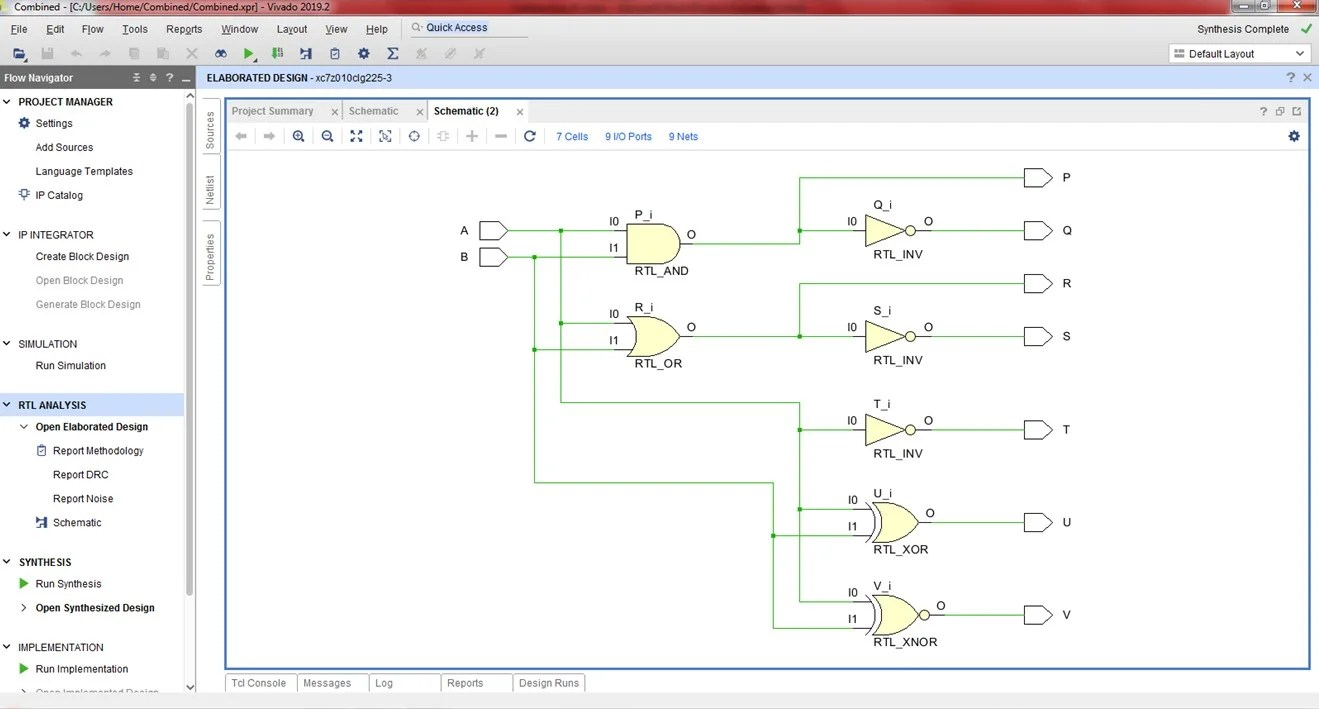## Simulation Waveforms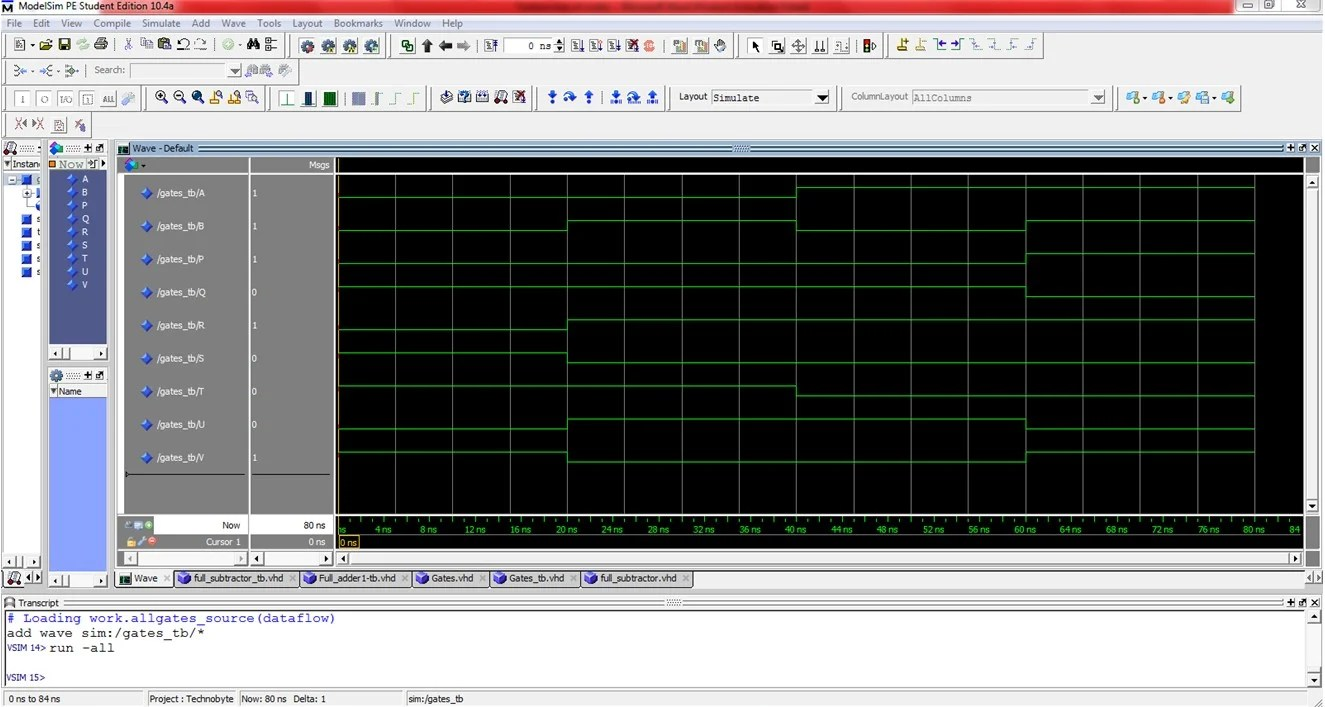We hope that this simple project of implementing all the logic gates in VHDL gave you an insight into VHDL programming. Next up, we will code some combinational circuits. If you have any queries, feel free to reach out to us in the comments below!

Edit: Post updated with the testbench, RTL Schematic, and Simulation Waveform by Deepak Joshi.

This site uses Akismet to reduce spam. Learn how your comment data is processed.